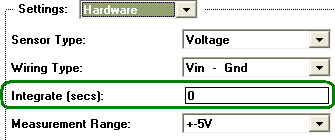Hidden Hidden Hidden Hidden
 SAMPLE RATE VS. INTEGRATION VS. NOISE REFERENCE > ADVANCED TECHNIQUES > What is Integration? Within the world of instruNet, the term "Integration" refers to when an A/D converter averages an incoming analog signal over a specified period of time. The Integrate field is set in the Hardware dialog, pictured below, for each channel, in units of seconds (e.g. 0.016 seconds). This is used to reduce noise, which is a fast up and down random signal added to the signal of interest. Integration, in some cases, enables one to average this random additional signal to a zero contribution, when the noise has an equal number of variations below and above the signal of interest. Your noise goes up when your integration time goes down. To see this, set different integration times (e.g. 0.016, 0.001, 0.0) and watch how much the displayed reading varies when the incoming signal is constant.Integration Limits Sample Rate For each instruNet i2x0/i60x controller, the sample rate (samples per second per channel) is limited by the sum of the Integration times used by the analog input channels. More specifically, the maximum sample period per channel (sample period = 1 / sample rate) is the sum of the integration times 1.2 (computer needs additional 20% time to manage other activities). For example, 4 channels digitized at 100s/sec/ch would have a sample period of 10msec/ch (0.01 seconds in between each point within one digitized channel), and the maximum total integration time (i.e. the sum of the integrations for each of the 4 channels) minus 20% would be 8ms. Therefore, if each of the 4 channels had the same integration time, the maximum integration time for this sample rate would be 2ms (8ms/4) per channel. Here's another example. If you want to digitize 8 channels at 10 sample per second per channel (s/sec/ch), set your integration time to 0.01 seconds for each of the 8 channels to establish an 100ms/ch minimum sample period (same as 10s/sec/ch max sample rate). One could then reduce integration 8x to get a sample rate that is 8x faster. How to View/Adjust Integration Times To view/adjust channel integration times, open the instruNet World NETWORK page, click on an analog input channel and select HARDWARE settings (or click QUICK SETUP button in NETWORK page), and view/adjust the Integrate field (units are seconds), illustrated above. Typical values are 0.01666 (one 60Hz power line cycle), 0.02 (one 50Hz power line cycle), 0.001 (1ms), and 0 (no integration). Viewing Maximum Sample Rate To view maximum sample rate, click RECORD tab at bottom of window, click SETUP button, set sample rate to 1e9 (beyond capability), click OK, click START digitize, view sample rate at top of window (i.e. maximum samples digitized per second per channel) and click STOP button. Also, one can re-enter SETUP dialog to see new maximum sample rate.